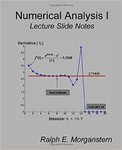## Faculty Book Gallery#### Title

Numerical Analysis I: Lecture Slide Series (Volumes 1 & 2)

#### Description

These Lecture Slide Notes have been used over the past several years for a two-quarter graduate level sequence in numerical analysis. Part 1 covers introductory material on the Nature of Numerical Analysis, Root Finding Techniques, Polynomial Interpolation, Derivatives, and Integrals. Part 2 covers Ordinary Differential Equations and Numerical solutions to Linear Systems of Equations. Each slide stands alone to encapsulate a complete concept, algorithm, or theorem using a combination of equations, graphs, diagrams, illustrative tableaus, and comparison tables. The explanatory notes are placed directly below each slide in order to reinforce and give additional insight into the particular numerical technique or concept illustrated in the slide. Students have found this “Lecture Slide Note” format to be extremely useful in reviewing the concepts in preparation for an exam. This format is convenient for self-study; it covers the subject matter in a concise and easily accessible form using many visualizations. The Table of Contents serves to organize the slides in terms of the main numerical analysis topics covered and gives a complete list of slide Titles and their page numbers. A selection of Illustrative MatLab scripts is given in Appendix A. Finally, references to a number of standard text books are given, but there has been no attempt to make an exhaustive bibliography.

978-1500946623

2014

#### Publisher

CreateSpace Independent Publishing Platform

#### Disciplines

Applied Mathematics | Numerical Analysis and ComputationCOinS